Next: Fourier transformations Up: 1: WHAT IS A Previous: 1: WHAT IS A

## Functions with discontinuities

Let us consider the functions listed in the left column of Table 1.
 5|c|Table 1: Functions and discontinuities function order A0 A1 A2 H(t) 11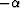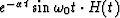1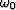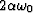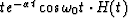1 1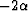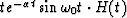2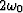2 2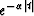1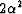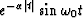1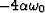All these functions have one general property in common: a discontinuity (a jump of derivatives) at the point t=0. The simplest example of a discontinuity is given by the step function H(t) (Figure). It has a discontinuity of order 0. We observe the same discontinuity for the second function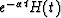. One can find the orders of the discontinuities of the other functions in column 2. In columns 3 etc., the amplitudes of derivative jumps are given:

 Ak=f(k)(0+)-f(k)(0-) (1)

where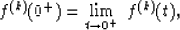Definition: The function f(t) has a discontinuity of order r at the point t=0, if for alland for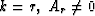.Values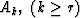are called amplitudes of order k.Next: Fourier transformations Up: 1: WHAT IS A Previous: 1: WHAT IS A
Stanford Exploration Project
1/13/1998